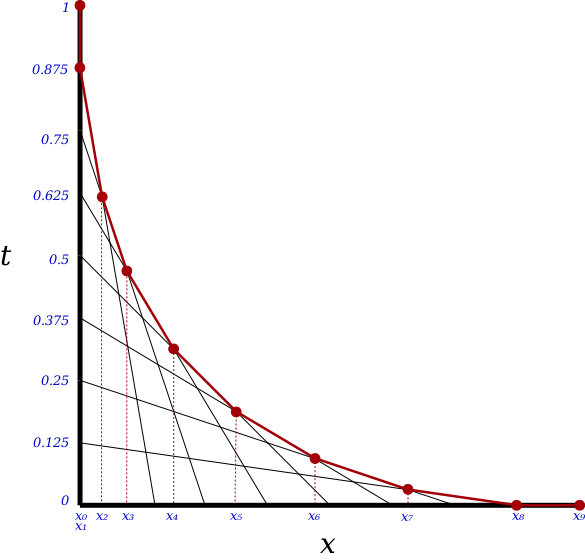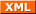#tianjara.net |Andrew Harvey's Blog

### Entries from January 2010.

22nd January 2010

So I've started reading a book about networks, and to complement this I've been taking a closer look at my network traffic in Wireshark (really great tool, by the way.).

So I pick an ftp site that I know, ftp://download.nvidia.com/ and see what happens in Wireshark when I visit it in Firefox. At the FTP application level this is what happens,

ftpsite to me: 220 spftp/1.0.0000 Server [69.31.121.43]\r\n
me to ftpsite: USER anonymous\r\n
ftpsite to me: 331 Password required for USER.\r\n
me to ftpsite: PASS mozilla@example.com\r\n
ftpsite to me: 230- \r\n
230- ---------------------------------------------------------------------------\r\n
230- WARNING:  This is a restricted access system.  If you do not have explicit\r\n
230-           permission to access this system, please disconnect immediately!\r\n
230 ----------------------------------------------------------------------------\r\n


But Firefox does not disconnect. So I did some more research and I found no references to "anonymous" users in either RFC 959 (FTP) or RFC 3659 (extensions to FTP). (Though there are references in latter RFCs, see RFC 2228).

The thing I find disconcerting is that I don't think I have "explicit permission" to access this system. I (or rather Firefox) just guessed a username and password and they happened to let me in (what if I guessed a different username and password that wasn't anonymous and it let me in?). If the RFC specified that a user of anonymous requires no password, or any password, then I would assume that the FTP server is granting me permission, but I assume rather people just started using anonymous as the user and it caught on...

The real problem here is that there are laws which govern such areas, and it doesn't help that that I don't understand what PART 6 - COMPUTER OFFENCES of the CRIMES ACT 1900 (NSW) is saying.

Tags: computing, law.
19th January 2010

How weird is this, just recently when I started up my computer lots of stuff was broken, no audio (and /proc/asound/cards was empty, normally it has "0 [Intel          ]: HDA-Intel - HDA Intel\nHDA Intel at 0xfa100000 irq 22"), libsensors weren't reporting any values (eg. no CPU temp reported), eth0 dissapeared from NetworkManager, and probably a host of other things that I didn't notice. Restarting didn't fix it.

Well long story short, it turns out that everything magically fixed when I unplugged a USB hard drive that was plugged in. I had seen a lot of concerning messages sent to /var/log/messages from the kernel about it,

Jan 19 09:45:00 host kernel: [  564.100026] usb 1-3: reset high speed USB device using ehci_hcd and address 2 Jan 19 09:45:00 host kernel: [  564.237716] sd 8:0:0:0: [sdd] Unhandled error code Jan 19 09:45:00 host kernel: [  564.237719] sd 8:0:0:0: [sdd] Result: hostbyte=DID_ABORT driverbyte=DRIVER_OK

that repeated every so often, but I never thought that a dodgy USB device would break the kernel from doing some of its job.

Tags: computing.
7th January 2010

This is the kind of post that I originally envisioned that I would post about when I started this blog. But after trying to complete this post I realised why I don't do this very much, because I can't always solve the problems I come up with. Anyway...

You can generate a funky kind of grid by taking a Cartesian coordinate system, joining lines from $(0, t)$ to $(t, 0)$ for some values t. Here are some examples,If you draw lots of lines you get something like,

[caption id="attachment_982" align="aligncenter" width="500" caption="Generated using code at http://github.com/andrewharvey/cairomisc/blob/bd41c8feb1beba38849878aed566c6f45a70856b/curved_grid_simple.pl"][/caption]

This is also what you get if you take a bunch of horizontal lines from $x = 0$ to $x = 1$ (where the horizontal lines are equally spaced above each other), and take all the endpoints from the line $x = 1$ and rotate them $90^\circ$ about the point $1, 0$.

The thing I was interested in was as you draw more and more of these lines it looks like a curve emerges on the boundary. I imagined that if you drew infinitely many lines like these you would get a nice smooth curve. I want to know what is the formula for that curve. But as I started to try to work it out, it didn't seem so simple.

I tried a lot of approaches, none of which seemed to work. So after a few initial set backs I tried to take a parametric approach taking t to be a value between 0 and 1 where this t indicates the line with start point $(0, t)$. The point on the curve for this t is some point on that line. I tried to get that point via the intersection with the next line, ie. the point on this line that is also on the curve is between the intersection of that line and the line for $t + \phi$ and $t - \phi$ for some really small $\phi$. But when I tried this approach as you make $\phi$ zero, then we get infinitely many points of intersection.

That didn't work so easy but then I realised that if the point is on this line then (although I have not proved this but it seems obvious from the picture) that I have the gradient.

So all those lines as shown above have equation $y = \frac{-t}{\left ( 1 - t \right )}x + t$. (Except for t = 1 where we'll just use a y value of 1). We can use this same t to define a point on the curve (which I call $f$ from here on) parametrically. So I assumed that the gradient of $f$ is given as $f'(t) = \frac{-t}{1-t}$. But now I'm not so sure that I have enough rigour here.

But then I got stuck again. I can try to go some integrals but this won't work because you don't know the relation between increasing t and the length along the curved you have moved. As you could have two different parametric functions which both have the same derivative function (ignoring the +c constant that disappears when you differentiate), just knowing the function defining the derivative of $f$ parametrically won't tell me the equation of the original curve.

Moving on I now tried to calculate the area under the curve. I could partition it like how a Riemann integral is done.We can easily calculate the area of any of these trapezoids (bounded by red). $A = \frac{x_n - x_{(n-1)}}{2}(y_{x_n} + y_{x_{(n-1)}})$. We can get the x values by finding the point of intersection of the 2 lines that intersect at that x (and have the largest y value if there are several points of intersection for that x). Each line for some value t will have a point of intersection of the line before and after it (based on the t value). When I say the area of t = some number, I mean the area of the trapezoid starting with the intersection of the previous t line and ending with the intersection of the next t line. So the area of t = 1 is zero (because x0 and x1 are the same). The diagram above has $\phi = 0.125$. So,

Point A is the intersection of $y = \frac{-t}{\left ( 1-t \right )}x + t$ and $y = \frac{- \left ( t + \phi \right )}{1-\left ( t + \phi\right )}x + t + \phi$, which is,

$x_A = (1-t)(1-t-\phi)$ $y_A = t(t+\phi)$

Point B is the intersection of $y = \frac{-t}{\left ( 1-t \right )}x + t$ and $y = \frac{- \left ( t - \phi \right )}{1-\left ( t - \phi\right )}x + t - \phi$, which is,

$x_B = (1-t)(1-t+\phi)$ $y_B = t(t-\phi)$

So the area of this trapezoid is $\frac{x_B - x_A}{2}(y_A + y_B)$, which is $2t^2\phi(1-t)$

But then I got stuck here. I can compute a value for the approximate area.

phi = 0.0001;
area = 0;
for (t = 1; t > 0; t -= phi) {
area += 2*t*t*phi*(1-t);
}
print area;


Which gives a value very close to 1/6, and if I integrate that area equation for t = 0..1 you get $\frac{1}{6}\phi$. But I don't want the area, I want the formula that defines the area from x = 0 to some value x so that I can then differentiate this to get the equation of the original curve. So this is where I give up, and leave this for another day. If you work it out please post in the comments!

Oh and there is some rough code I wrote to make those images here. And a nice animation too.

Tags: mathematics.
4th January 2010

What happens when you mix a service like AustLii with version control system like Git with a wiki like editing system, and deliver it to the people through the web?

Well I haven't tried, but it sounds like a good idea. You get a service that,

• allows anyone to propose changes to laws (and work on branches) or draft and new laws, and
• keeps track of the law and when it was changed (and which politicians/parties introduced those changes, who voted for them, etc...).
Tags: law, politics.